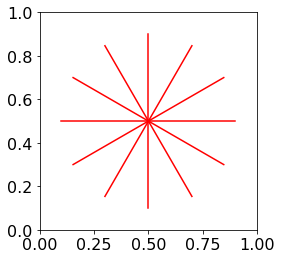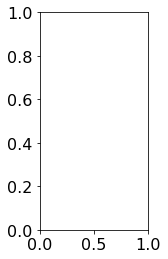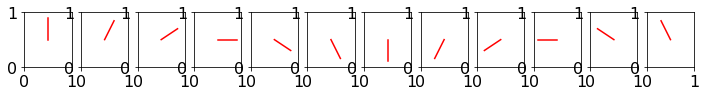# Animations¶

In this reading, we'll animate a clock hand going in circles. First, let's write a function that draws a hand for a specific time (no animation). We'll use sin and cos (which use radians!), and assume that the hand pointing directly up is 0 degrees.

In :
%matplotlib inline

In :
from math import sin, cos, pi
import matplotlib.pyplot as plt
plt.rcParams["font.size"] = 16

In :
fig, ax = plt.subplots()
ax.set_aspect("equal")

def draw_hand(area, time):
# average range to get center of plot
x1 = (area.get_xlim() + area.get_xlim()) / 2
y1 = (area.get_ylim() + area.get_ylim()) / 2
time %= 12 # 13 o'clock is the same as 1 o'clock (treat 12 as 0)
percent = time / 12
radians = percent * 2 * pi

hand_length = 0.4
x2 = x1 + sin(radians) * hand_length
y2 = y1 + cos(radians) * hand_length
hand = plt.Line2D((x1, x2), (y1, y2), color="r")

for time in range(12):
draw_hand(ax, time)There are all the hand positions, shown in one plot. Don't worry to much if you don't completely understand the trig details. Do notice we're creating a Line2D object and adding it to the are with ax.add_artist(...). This may also be new to you: ax.set_aspect("equal"). It means that an interval on the x-axis will take up the same space as the same interval on the y-axis. In contrast, the following plot uses twice as much space to go from 0 to 1 on the y-axis as on the x-axis, so the aspect ratio is 2.

In :
fix, ax = plt.subplots()
ax.set_aspect(2)Let's create 12 subplots, with each time position.

In :
fix, axes = plt.subplots(ncols=12, figsize=(12,1))

for i in range(12):
ax.set_aspect(1)
draw_hand(axes[i], time=i)To create an animation, you could imagine quickly flipping through the above still images. When used this way, we would call those still images frames of our animation.

FuncAnimation objects in matplotlib help us automatically generate the frames for an animation, then stitch them together into a video:

In :
from matplotlib.animation import FuncAnimation


We'll create a new animation with this: FuncAnimation(OUR_FIGURE, SOME_FUNCTION_REFERENCE).

SOME_FUNCTION_REFERENCE should be a reference to some function that can be repeatedly called by the FuncAnimation. The function should take as a parameter an integer describing the current frame number, then it should draw that frame on the figure.

In :
fig, ax = plt.subplots()
ax.set_aspect("equal")

def draw_frame(frame_num):
t = frame_num / 4
print(f"For frame {frame_num}, draw hand at time {t}")
draw_hand(area=ax, time=t)

fa = FuncAnimation(fig, draw_frame) # no (), so we're not calling draw_frame!
print("created FuncAnimation, now let's create an HTML5 video with it")
html = fa.to_html5_video()
print("done creating the video, so let's close the figure")
plt.close(fig)

created FuncAnimation, now let's create an HTML5 video with it
For frame 0, draw hand at time 0.0
For frame 0, draw hand at time 0.0
For frame 1, draw hand at time 0.25
For frame 2, draw hand at time 0.5
For frame 3, draw hand at time 0.75
For frame 4, draw hand at time 1.0
For frame 5, draw hand at time 1.25
For frame 6, draw hand at time 1.5
For frame 7, draw hand at time 1.75
For frame 8, draw hand at time 2.0
For frame 9, draw hand at time 2.25
For frame 10, draw hand at time 2.5
For frame 11, draw hand at time 2.75
For frame 12, draw hand at time 3.0
For frame 13, draw hand at time 3.25
For frame 14, draw hand at time 3.5
For frame 15, draw hand at time 3.75
For frame 16, draw hand at time 4.0
For frame 17, draw hand at time 4.25
For frame 18, draw hand at time 4.5
For frame 19, draw hand at time 4.75
For frame 20, draw hand at time 5.0
For frame 21, draw hand at time 5.25
For frame 22, draw hand at time 5.5
For frame 23, draw hand at time 5.75
For frame 24, draw hand at time 6.0
For frame 25, draw hand at time 6.25
For frame 26, draw hand at time 6.5
For frame 27, draw hand at time 6.75
For frame 28, draw hand at time 7.0
For frame 29, draw hand at time 7.25
For frame 30, draw hand at time 7.5
For frame 31, draw hand at time 7.75
For frame 32, draw hand at time 8.0
For frame 33, draw hand at time 8.25
For frame 34, draw hand at time 8.5
For frame 35, draw hand at time 8.75
For frame 36, draw hand at time 9.0
For frame 37, draw hand at time 9.25
For frame 38, draw hand at time 9.5
For frame 39, draw hand at time 9.75
For frame 40, draw hand at time 10.0
For frame 41, draw hand at time 10.25
For frame 42, draw hand at time 10.5
For frame 43, draw hand at time 10.75
For frame 44, draw hand at time 11.0
For frame 45, draw hand at time 11.25
For frame 46, draw hand at time 11.5
For frame 47, draw hand at time 11.75
For frame 48, draw hand at time 12.0
For frame 49, draw hand at time 12.25
For frame 50, draw hand at time 12.5
For frame 51, draw hand at time 12.75
For frame 52, draw hand at time 13.0
For frame 53, draw hand at time 13.25
For frame 54, draw hand at time 13.5
For frame 55, draw hand at time 13.75
For frame 56, draw hand at time 14.0
For frame 57, draw hand at time 14.25
For frame 58, draw hand at time 14.5
For frame 59, draw hand at time 14.75
For frame 60, draw hand at time 15.0
For frame 61, draw hand at time 15.25
For frame 62, draw hand at time 15.5
For frame 63, draw hand at time 15.75
For frame 64, draw hand at time 16.0
For frame 65, draw hand at time 16.25
For frame 66, draw hand at time 16.5
For frame 67, draw hand at time 16.75
For frame 68, draw hand at time 17.0
For frame 69, draw hand at time 17.25
For frame 70, draw hand at time 17.5
For frame 71, draw hand at time 17.75
For frame 72, draw hand at time 18.0
For frame 73, draw hand at time 18.25
For frame 74, draw hand at time 18.5
For frame 75, draw hand at time 18.75
For frame 76, draw hand at time 19.0
For frame 77, draw hand at time 19.25
For frame 78, draw hand at time 19.5
For frame 79, draw hand at time 19.75
For frame 80, draw hand at time 20.0
For frame 81, draw hand at time 20.25
For frame 82, draw hand at time 20.5
For frame 83, draw hand at time 20.75
For frame 84, draw hand at time 21.0
For frame 85, draw hand at time 21.25
For frame 86, draw hand at time 21.5
For frame 87, draw hand at time 21.75
For frame 88, draw hand at time 22.0
For frame 89, draw hand at time 22.25
For frame 90, draw hand at time 22.5
For frame 91, draw hand at time 22.75
For frame 92, draw hand at time 23.0
For frame 93, draw hand at time 23.25
For frame 94, draw hand at time 23.5
For frame 95, draw hand at time 23.75
For frame 96, draw hand at time 24.0
For frame 97, draw hand at time 24.25
For frame 98, draw hand at time 24.5
For frame 99, draw hand at time 24.75
done creating the video, so let's close the figure


Based on those prints, it looks like draw_frame is being called many times! FuncAnimation can do this because we gave it a reference to draw_frame. FuncAnimation is class, but logically, it's a little like this function:

def animate(some_func):
for frame in range(100):
some_func(frame)

animate(draw_frame)


We did see "created FuncAnimation, now let's create an HTML5 video with it" is printed after the FuncAnimation is created, so one difference is that FuncAnimation doesn't call some_func until it has to (that is, when it's time to generate the video with .to_html5_video()).

The HTML we got back contains a video tag, as we can see if we look at a slice with the first few hundred characters:

In :
html[:300]

Out:
'<video width="432" height="288" controls autoplay loop>\n  <source type="video/mp4" src="data:video/mp4;base64,AAAAHGZ0eXBNNFYgAAACAGlzb21pc28yYXZjMQAAAAhmcmVlAAC4uG1kYXQAAAKtBgX//6ncRem9\n5tlIt5Ys2CDZI+7veDI2NCAtIGNvcmUgMTU3IHIyOTY5IGQ0MDk5ZGQgLSBILjI2NC9NUEVHLTQg\nQVZDIGNvZGVjIC0gQ29weWxlZnQgMjAwMy0y'

We could save that in an HTML file, or even just embed it in our notebook:

In :
from IPython.core.display import HTML
HTML(html)

Out:

Well, it's an animation, but new hands keep getting created. We really wanted it to look like one hand was moving. We can use ax.cla() at the start of each frame to make sure the hands from previous frames go away.

In :
fig, ax = plt.subplots()
ax.set_aspect("equal")

def draw_frame(frame_num):
ax.cla()
t = frame_num / 4
draw_hand(area=ax, time=t)

fa = FuncAnimation(fig, draw_frame)
html = fa.to_html5_video()
plt.close(fig)

HTML(html)

Out:

What if we want the video to be smoother? We need more frames (default is 100), shown at greater frequency (default interval is 200 milliseconds), with smaller changes between frames (we used t = frame_num / 4, so the hand moves a quarter hour for each frame).

To make it twice as smooth while maintaining the same speed, let's use 200 frames, 100 millisecond intervals, and move the hand one eighth each time.

In :
fig, ax = plt.subplots()
ax.set_aspect("equal")

def draw_frame(frame_num):
ax.cla()
t = frame_num / 8
draw_hand(area=ax, time=t)

fa = FuncAnimation(fig, draw_frame, frames=200, interval=100)
html = fa.to_html5_video()
plt.close(fig)

HTML(html)

Out:

That's a higher quality video, but it took longer to generate. It is recommended to first get your animation working with course-grained animation while debugging, then make it smooth as a last step.

In [ ]: